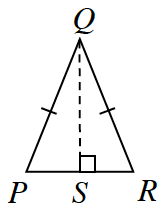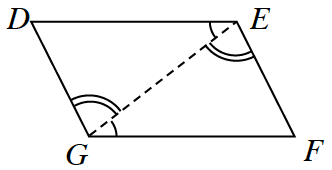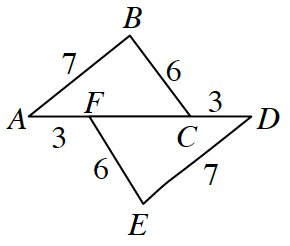### Home > CC4 > Chapter 8 > Lesson 8.1.1 > Problem8-11

8-11.

For each pair of triangles below, determine if the triangles are congruent. If the triangles are congruent, complete the congruence statement and state the congruence property. Otherwise, explain why you cannot conclude that the triangles are congruent. Homework Help ✎

1. $ΔSQP ≅ Δ\text{____}$$ΔSQR$; HL≅ (Remember $\overline{QS} = \overline{SQ}$, Reflexive)

1. $ΔEDG ≅ Δ\text{____}$$ΔGFE$; ASA≅ (Remember $\overline{GE} = \overline{EG}$ Reflexive)

1. $ΔABC ≅ Δ\text{____}$$ΔDEF$; $\text{SSS}≅$